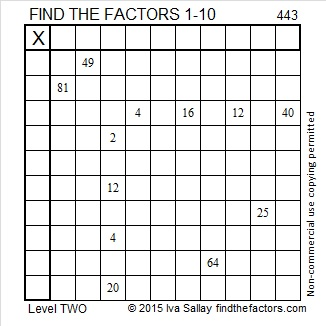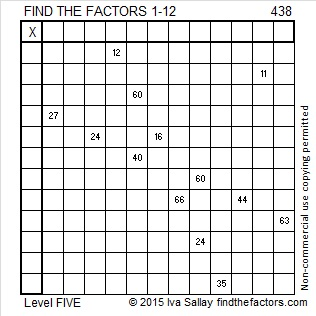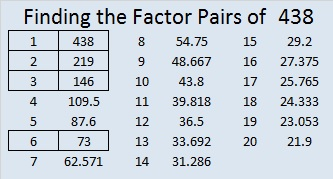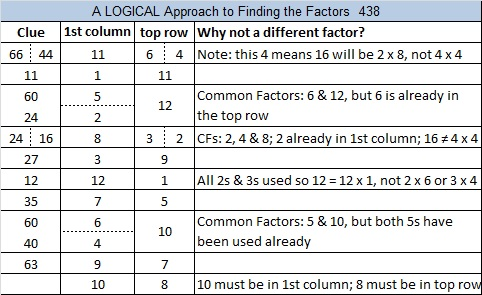# 443 and Level 2

Most people can tell just by looking that 443 cannot be evenly divided by 2, 3, or 5. Could it possibly be a prime number? Scroll down past the puzzle to know for sure.Print the puzzles or type the factors on this excel file: 10 Factors 2015-03-30

• 443 is a prime number.
• Prime factorization: 443 is prime.
• The exponent of prime number 443 is 1. Adding 1 to that exponent we get (1 + 1) = 2. Therefore 443 has exactly 2 factors.
• Factors of 443: 1, 443
• Factor pairs: 443 = 1 x 443
• 443 has no square factors that allow its square root to be simplified. √443 ≈ 21.0476How do we know that 443 is a prime number? If 443 were not a prime number, then it would be divisible by at least one prime number less than or equal to √443 ≈ 21.0476. Since 443 cannot be divided evenly by 2, 3, 5, 7, 11, 13, 17, or 19, we know that 443 is a prime number.# 438 and Level 5

The sum of the digits of 438 is 4 + 3 + 8 = 15. The sum of the digits of its prime factorization (2 x 3 x 73) is 2 + 3 + 7 + 3 = 15. Since the two sums are equal, 438 is the 18th Smith number.Print the puzzles or type the factors on this excel file: 12 Factors 2015-03-23

• 438 is a composite number.
• Prime factorization: 438 = 2 x 3 x 73
• The exponents in the prime factorization are 1, 1, and 1. Adding one to each and multiplying we get (1 + 1)(1 + 1)(1 + 1) = 2 x 2 x 2 = 8. Therefore 438 has exactly 8 factors.
• Factors of 438: 1, 2, 3, 6, 73, 146, 219, 438
• Factor pairs: 438 = 1 x 438, 2 x 219, 3 x 146, or 6 x 73
• 438 has no square factors that allow its square root to be simplified. √438 ≈ 20.9284